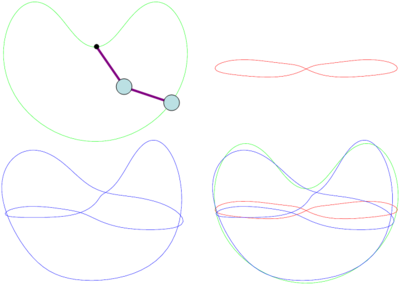# Periodic orbits

(diff) ← Older revision | Latest revision (diff) | Newer revision → (diff)Shadowing of two periodic orbits (red and green) leads to a longer one (blue).

Apparently complex dynamics can originate from a small set of dynamically important recurring patterns. The image to the right shows two recurring patterns (green,red) that exist for the 'double pendulum', where a second pendulum is attached to the weight of a first pendulum. (A video showing periodic orbits of the double-pendulum can be found HERE.) As time progresses, the position of the lower pendulum traces out the lines shown, (x,y)=(position of lower pendulum). As the line is a closed loop, it traces out a repeating pattern - a 'periodic orbit'.

Observe that the longer orbit (blue) can be considered to be constructed from 'shadowing' of the two simpler orbits. It is possible to understand a lot about the dynamics by finding the short periodic orbits. These short orbits we might call our 'alphabet', out of which infinitely many 'words' may be constructed, i.e. an infinite number of longer orbits.

An excellent (graduate level) free text on periodic orbits can be found at chaosbook.org.

### Periodic orbits in pipe flow

In this case, the 'state' of the system corresponds to the speed and direction of travel of the fluid at every position in the flow. By listing the velocity at every point in space we form a state-vector, a=(a1,a2,a3,a4,...). As there are infinitely many points, the dimension (length) of a is formally infinite. However, for 'dissipative' systems, the dynamics typically lives on a space of much smaller dimension, like a 2D-surface within 3D space. This is because the dynamics avoids unrealistic states, and is attracted to a more typical subset of states. To visualise the typical dynamic pathways, it is necessary to project the state onto a shorter, more meaningful vector, e.g. (x,y)=(energy,dissipation).

The following video shows a selection of periodic orbits in pipe flow, visualised in terms input energy driving the flow (I), dissipation (D) and kinetic energy (E) of the motion (relative to their laminar (non-turbulent) values):

(The setup is pipe flow with flow rate measured by Re=DU/nu=2500; D=diameter, U=mean axial speed, nu=kinematic viscosity.)

The next video shows turbulent flow in the lab frame. Regions of flow slower than the mean flow are indicated in blue, called 'streaks'. Rotating 'vortex structures' are indicated in yellow. To simplify analysis a 4-fold rotational symmetry has been imposed. This does not significantly affect statistical properties of the flow, e.g. the turbulent friction factor.

The next video shows the same flow viewed in a moving frame where it is possible to see recurring patterns, i.e. periodic orbits. The speed at which the frame moves is different for every periodic orbit. To determine this speed automatically we use the Method of slices. The periodic orbit itself was found using the Newton-Krylov method.

Periodic orbits exist that encompass the broad range of observed flow patterns. The following video shows an example of a periodic orbit that is representative of a spatially localised patch of turbulence, often called a turbulent 'puff':